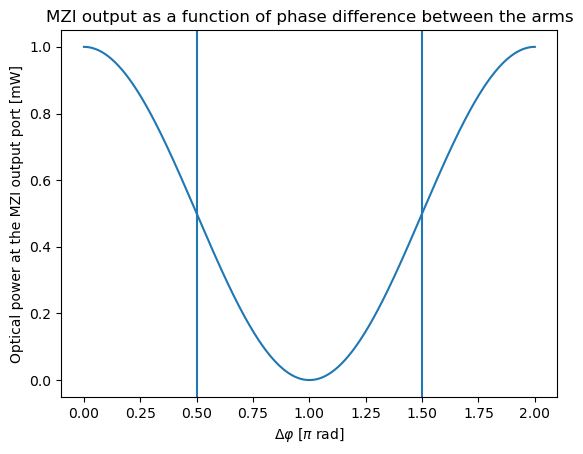# 1.1. Introduction¶

A Mach-Zehnder modulator (MZM) is a device used to achieve amplitude modulation. It is based on an interferometric structure, called a Mach-Zehnder interferometer (MZI).Fig. 1.4 Schematic representation of a Mach-Zehnder interferometer (MZI).

In an MZI, the incoming optical wave is first split in two arms using a Y-splitter and is then recombined by a second Y-splitter. When the two beams are recombined, two different scenarios can take place:

1. If the two separate paths have equal optical length, the split beams are in-phase when they recombine and constructive interference is achieved.
2. If the optical length of the two paths is different, the split beams will have different relative phase when they recombine. This gives rise to destructive interference.

Therefore, depending on the relative phase difference acquired by the split beams while traveling along the two separate paths, the light is recombined more or less efficiently. In the following image, the transmission of an MZI is plotted as a function of the phase difference $$\Delta\Phi$$.Fig. 1.5 Transmission of an MZI as a function of the phase difference $$\Delta\Phi$$ between the arms.

To control the relative phase shift between the split beams, a phase shifter is placed on each of the two arms of the interferometer. When a voltage is applied to the phase shifter, the optical path length is altered due to the electro-optic effect. If the relative phase difference between the two arms of the interferometer is equal to $$\pi$$, destructive interference takes place when the beams recombine and a minimum of intensity is achieved. If the phase difference is equal to 0, constructive interference gives rise to a maximum of intensity. Switching between these two states allows us to achieve amplitude modulation.Fig. 1.6 Schematic representation of a Mach-Zehnder modulator (MZM) with a phase shifter on each arm.

The MZM in this application example will, by design, introduce a path length difference of $$\pi/2$$ between the two arms to operate in the area of maximum slope in transmission of the MZI.

In real life, due to typical fabrication errors, the actual length of the MZI arms differs slightly from the design specifications. To fine-tune the optical length, a heated waveguide section is added after the Y-splitter, so that the operating point can be maintained as wavelength and operation temperature vary. Due to the strong thermo-optic effect in silicon, heaters are particularly well suited for this job as they allow us to efficiently vary the optical length of the waveguide by changing the waveguide mode effective index, resulting in a change of the optical phase of the propagated light.Fig. 1.7 Schematic representation of a Mach-Zehnder modulator (MZM) with a phase shifter and a heater on each arm.

For more details on the design and the compact model of the heater we will use for the MZM, have a look at the documentation of the heater included in SiFab: Heaters.

In the next sections you will learn how to implement and simulate

• a phase shifter,
• a heated waveguide, and
• a Mach-Zehnder modulator.